# PSAT Math : How to find the probability of an outcome

## Example Questions

### Example Question #32 : How To Find The Probability Of An Outcome

Set S = {0, 1, 5, 9}, and set T = {2, 3, 4, 7}. If one number is chosen randomly from S and another is chosen randomly from T, what is the probability that the sum of these two numbers will be prime?

1/2

1/4

7/16

9/16

5/8

1/2

Explanation:

There are four possible numbers that can be chosen from S, and there are four that can be chosen from T. This means that there are 4 * 4, or 16, pairs of numbers that can be drawn from S and T. We need to find the sum of these pairs and determine if each sum is prime. Let's find the sum of the 16 pairs. Remember that a number is prime if it divisible only by itself and 1.

0 + 2 = 2, which is prime

0 + 3 = 3, prime

0 + 4 = 4, not prime

0 + 7 = 7, prime

1 + 2 = 3, prime

1 + 3 = 4, not

1 + 4 = 5, prime

1 + 7 = 8, not

5 + 2 = 7, prime

5 + 3 = 8, not

5 + 4 = 9, not

5 + 7 = 12, not

9 + 2=11, prime

9 + 3 = 12, not

9 + 4 = 13, prime

9 + 7 = 16, not

Of the sixteen pairs, 8 have a sum that equals a prime number. Thus, because each of these pairs has an equal chance of being drawn randomly, the probability that the sum will be prime is 8 out of 16, or 8/16 = 1/2.

### Example Question #31 : Probability

Judy is practicing to be a magician and has an ordinary deck of 52 playing cards, a regular 6-sided die, and a fair coin. What is the probability that Judy rolls a 6 on the die, then flips the coin head face up, and then draws a spade from the deck of cards?

11/12

2/25

1/48

3/16

1/4

1/48

Explanation:

We can find the individual probabilities of these three events occuring first.

P(rolling a 6) = 1/6

Now, to find the probability of rolling a 6 AND flipping a head AND drawing a spade, we must multiply the individual probabilities. So the answer is 1/6 * 1/2 * 1/4 = 1/48.

### Example Question #31 : Probability

A bag of marbles has 7 yellow marbles, 5 red marbles, 3 blue marbles, and 6 white marbles. What is the probability of choosing a yellow marble, putting it back and choosing a blue marble, and then NOT putting the blue marble back and picking a white marble?

2/3

8/31

3/17

1/9

1/70

1/70

Explanation:

There are a total of 7 + 5 + 3 + 6 = 21 marbles. The probability of picking a yellow marble is 7/21 = 1/3. Then we put it back and choose a blue marble with probability 3/21 = 1/7. We do NOT put this blue marble back, but then we grab for a white. The probability of picking a white is now 6/20 = 3/10, because now we are choosing from 20 marbles instead of 21. So putting it together, the probability of choosing a yellow marble, replacing it and then choosing a blue and a white, is 1/3 * 1/7 * 3/10 = 1/70.

### Example Question #131 : Statistics

In a regular 52-card deck, what is the probability of drawing three aces in a row, with replacement?

1/52 * 1/52 * 1/52

1/52 * 1/51 * 1/50

13/52 * 13/51 * 13/50

4/52 * 4/52 * 4/52

1/52 + 1/52 + 1/52

4/52 * 4/52 * 4/52

Explanation:

There are 4 aces in the 52 card deck, so the probability of drawing an ace is 4/52. Then we put this ace back in the deck and draw again. The probability of drawing an ace is again 4/52. Similarly, on the third draw, the probability of getting an ace is 4/52. So the probability of drawing an ace on the 1st draw AND the 2nd draw AND the 3rd draw is 4/52 * 4/52 * 4/52.

### Example Question #41 : Probability

A bag contains four blue marbles, eight red marbles, and six orange marbles. If a marble is randomly selected from the bag, what is the probability that the marble will be either red or orange?

7/9

1/3

5/9

2/3

2/5

7/9

Explanation:

The probability of an event is the ratio of the number of desired outcomes to the total number of possible outcomes. In this problem, the total number of outcomes is equal to the total number of marbles in the bag. There are four blue, eight red, and six orange marbles, so the total number of marbles is the sum of four, eight, and six, or eighteen.

We are asked to find the probability of choosing a marble that is either red or orange. This means we have to consider the number of marbles that are either red or orange. Because there are eight red and six orange, there are fourteen marbles that are either red or orange.

The probability is thus fourteen out of eighteen, because there are fourteen red or orange marbles, out of a total of eighteen marbles. We will need to simplify the fraction 14/18.

Probability = 14/18 = 7/9

### Example Question #41 : How To Find The Probability Of An Outcome

Two dice are rolled. Find the probability that the numbers sum to 4.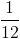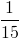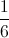Explanation:

The possible dice combinations that sum to 4 are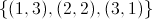.

The number of all possible dice combinations is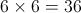. (6 numbers on each of the two dice.)

So the probability that the numbers sum to 4  =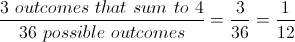### Example Question #141 : Statistics

Jackie is a contestant on a gameshow. She has to pick marbles out of a big bag to win various amounts of money. The bag contains a total of 200 marbles.

There are 100 red marbles worth $10 each, 50 blue marbles worth$20 each, 30 green marbles worth $50 each, 15 white marbles worth$100 each and 5 black marbles worth $1000 each. If she picks once, what percent chance does she have of picking a$1000 marble?

.05%

2.5%

3%

5%

2.5%

Explanation:

There are 5 black marbles worth \$1000 dollars out of the total of 200 marbles.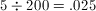or 2.5%

### Example Question #41 : Outcomes

What is the probability that you will pull out 3 diamonds in a standard deck without replacement.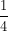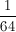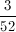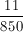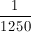Explanation:

There are 13 diamonds in a standard deck of 52 cards. So, you have a 13/52 (1/4) chance of getting a diamond; then a 12/51 (4/17) chance of pulling the next diamond; last, there is a 11/50 chance of getting the third diamond. When you combine probabilities, you multiply the individual probabilities together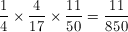### Example Question #41 : How To Find The Probability Of An Outcome

On Halloween there is a bowl of candy.  33 pieces are gum drops, 24 are candy corn, 15 are suckers and 28 are hard candies.  Without looking in the bowl, what are the chances that you pull a gum drop?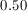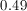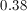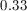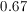Explanation:

Probability = # Gum Drops / # Total Number of Candies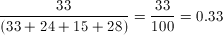### Example Question #41 : Probability

What is the probability of pulling out 4 diamonds in a standard deck of cards, without replacement?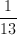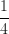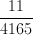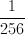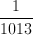There are 52 cards in a standard deck; 13 of them are diamonds. This means that there is a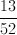chance that the first card you pick will be a diamond. The probability of pulling another diamond as your next card is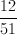; next diamond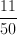; last diamond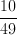.